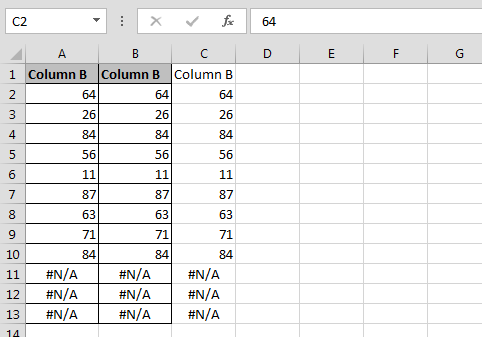# Change only the CurrentRegion of the ActiveCell to values using VBA in Microsoft Excel

In this article we will learn how to change only the current region of the active cell to values using VBA in Microsoft Excel.

Let’s take an example and understand how to change only the current region of the active cell to values.

How to change the value of current region?

We have data in Excel in which Column A contains some values and Column B also contains some values.  We are going to update the same value of column B in the current region.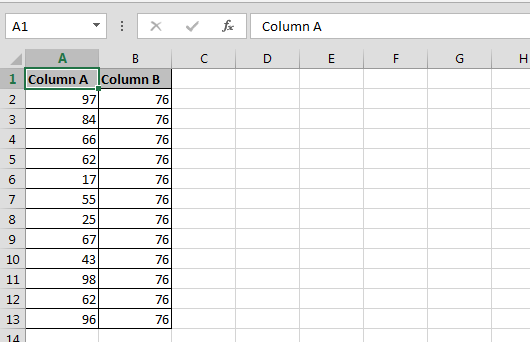• Press Alt+F11 key to open the Visual Basic Application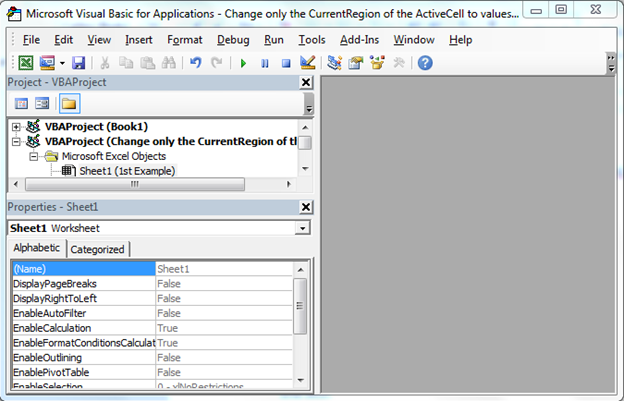• In VBA Project Double click on Sheet 1
• Enter the below given VBA Code

```Sub Values_2()

With ActiveCell.CurrentRegion

.Value = Range("B1:B10").Value

End With

End Sub
```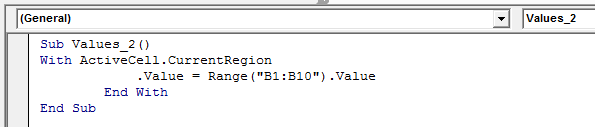• Select cell A1
• To run the code press F5 key
• Only first cell value will get updated in the data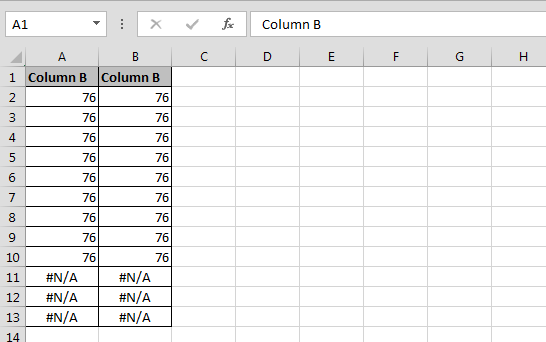Now let’s change the selection and see the results: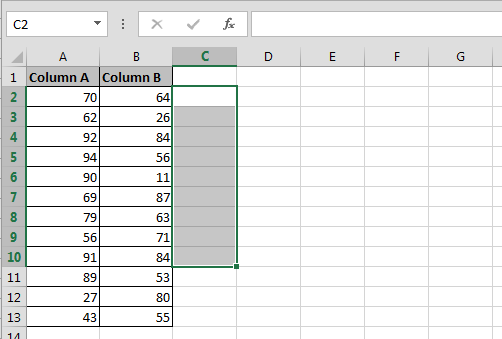• Select the range C1:C10
• Then press F5 to run the code